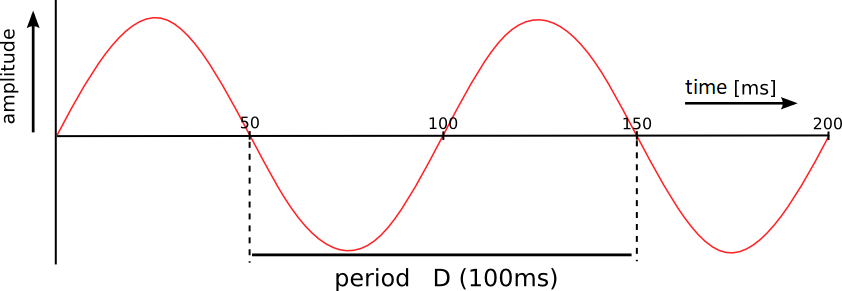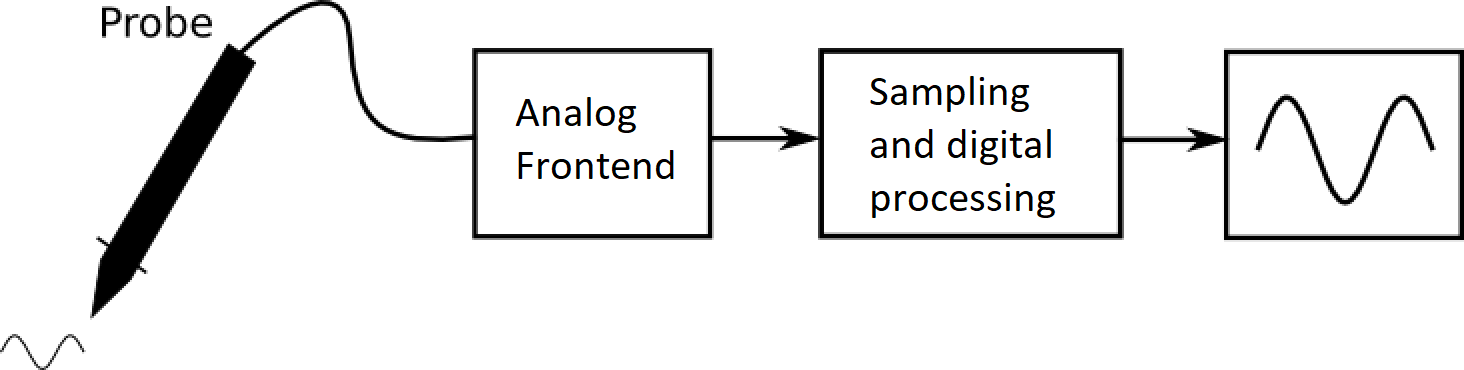Oscilloscopes are offered with different bandwidths and sample rates. Which bandwidth is most suitable? More is better of course, but higher bandwidth oscilloscopes are more expensive and the budget is not infinite.

## Frequencies and signals

Before we talk about bandwidth, we have to explain how signals are composed. When we talk about the frequency of an analog signal, we are always talking about the frequency of a sine wave. Figure 1 shows a sine wave. The picture shows two periods of the wave. The period is the time in which the sine wave goes up and down. The period and frequency are inversely proportional. The frequency can be calculated using the following formula: $$f = \frac{1}{D},$$ where $$f$$ is the frequency and $$D$$ the period. In figure 1 the period is 100 ms and the frequency is 10 Hz.Figure 1: Two periods of a sine wave are shown here.

## Non-sinusoidal signals

The sine wave is an important signal shape. Each periodic or repetitive signal consists of a list of sine waves. The picture below shows a square wave. Because a square wave has right angles, it is difficult to imagine that a square wave also consists of sine forms. However, the picture below shows that this is possible. The square wave consists of one base wave with sine waves on top of it with ever increasing frequencies, these are called the harmonics. The more harmonics, the better the signal looks like the square wave. A perfect square wave consists of an infinite number of harmonics and therefore does not exist in practice.Image 2: A square wave is made up of dozens of sine waves (Original).

When we talk about the frequency of a signal in this article, we are talking about the frequency of a sine wave, or the frequency of a harmonic.

## Bandwidth and sample rate

Now that we have briefly mastered the principles of harmonics and frequencies, we can go back to the oscilloscope. Figure 3 is a global diagram of a digital oscilloscope. The signal goes through the probe to the analog frontend. In the analog frontend the signal is amplified and filtered. Then it is sampled and converted to a digital signal. The digital signal is then displayed on the screen.Figure 3: Block diagram of the signal path in an oscilloscope.

The probe and the analog frontend each have a bandwidth. The bandwidth indicates to which frequency signals are still transmitted. Signals with a higher frequency than the bandwidth are attenuated. The transition between signals that are and are not passed is not direct. Signals with a frequency against the bandwidth are already attenuated. The picture below shows this clearly. The bandwidth is the frequency at which the attenuation of the signals is 30% (3dB).Figure 4: Bandwidth is the frequency band to the frequency at which the signal is attenuated by 3dB.

After the signal has been filtered by the analog frontend, the signal is sampled. With a fixed interval the signal is measured and digitized. The digitized signal is later displayed on the screen of the scope. A sine wave should be sampled several times per period to look good on the screen of the scope. Per period, the sine wave at least 5, but preferably 20 times to be sampled to look good on the screen. A sine wave with a frequency of 10Hz should be sampled 50 to 200 times per second.

## In practice

In practice, perfect sine waves are rarely measured, but also other signals, such as square waves. And when a signal is viewed, you often want to see what the signal looks like in detail. Therefore, the bandwidth of the scope should be much higher than the frequency of the signal to be measured. For example, when a motor is controlled with a 20 kHz square wave, a minimum bandwidth of 200 kHz is needed, but 500 kHz is better.

Always make sure that the probe's bandwidth is high enough. It is easiest if the probe's bandwidth is at least as high as the oscilloscope's bandwidth.

For our oscilloscopes the bandwidth is always mentioned, as is the sample rate in Mega Samples per second MS/s. In practice, with most oscilloscopes, the sample rate is much higher than the bandwidth.